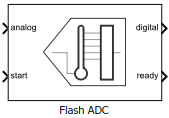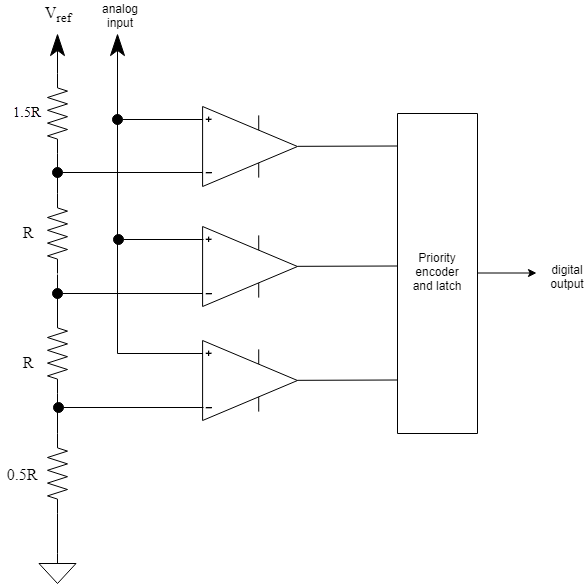• Library:
• Mixed-Signal Blockset / ADC / Architectures

•## Description

An N-bit flash ADC comprises of a resistive ladder that contains 2N resistors and 2N-1 comparators.The reference voltage of each comparator is 1 least significant bit (LSB) higher than the one below it in the ladder. As a result, all comparators below a certain point will have input voltage greater than the reference voltage, and a logic `1` output. All comparators above that point will have input voltage smaller than the reference voltage, and a logic `0` output. The output of 2N-1 comparators are passed through a priority encoder to produce the digital output. This encoding scheme is called thermometer encoding.

Since the analog input is applied to all the comparators at once, the flash ADC architecture is very fast. But the ADC has low resolution and high power requirements due to a large number of resistors required to implement the architecture.

## Ports

### Input

expand all

Data Types: `double`

External clock to start conversion, specified as a scalar. The analog to digital conversion process starts at the rising edge of the signal at the start port.

Data Types: `double`

### Output

expand all

Converted digital output signal, returned as scalar.

Data Types: `fixed point` | `single` | `double` | `int8` | `int16` | `int32` | `uint8` | `uint16` | `uint32` | `Boolean`

Determines whether the analog to digital conversion is complete, returned as a scalar.

Data Types: `double`

## Parameters

expand all

### Configuration

Number of physical output bits, specified as a unitless positive real integer in the range `[1, 26]`. Number of bits determines the resolution of the ADC.

#### Programmatic Use

• Use `get_param(gcb,'NBits')` to view the current Number of bits.

• Use `set_param(gcb,'NBits',value)` to set Number of bits to a specific value.

Data Types: `double`

ADC dynamic range, specified as a 2-element row vector in volts.

#### Programmatic Use

• Use `get_param(gcb,'InputRange')` to view the current Input range (V).

• Use `set_param(gcb,'InputRange',value)` to set Input range (V) to a specific value.

Data Types: `double`

Select to connect to an external start conversion clock. By default, this option is selected. If you deselect this option, a Sampling Clock Source block inside the Flash ADC is used to generate the start conversion clock.

Frequency of internal start conversion clock, specified as a positive real scalar in hertz. Conversion start frequency (Hz) determines the rate of the ADC.

#### Dependencies

This parameter is only available when Use external start clock is not selected.

#### Programmatic Use

• Use `get_param(gcb,'StartFreq')` to view the current value of Conversion start frequency (Hz).

• Use `set_param(gcb,'StartFreq',value)` to set Conversion start frequency (Hz) to a specific value.

Data Types: `double`

RMS aperture jitter added as an impairment to the start conversion clock, specified as a real nonnegative scalar in seconds. Set RMS aperture jitter value to zero if you want a clean clock signal.

#### Dependencies

This parameter is only available when Use external start clock is not selected.

#### Programmatic Use

• Use `get_param(gcb,'StartClkJitter')` to view the current value of RMS aperture jitter (s).

• Use `set_param(gcb,'StartClkJitter',value)` to set RMS aperture jitter (s) to a specific value.

Data Types: `double`

Clock edge type that triggers the output update:

• `Rising edge` — the output is updated with the rising edge of the clock signal.

• `Falling edge` — the output is updated with the falling edge of the clock signal.

• `Either edge` — the output is updated with both the rising and the falling edge of the clock signal.

#### Programmatic Use

• Use `get_param(gcb,'Trigger')` to view the current Edge trigger type.

• Use `set_param(gcb,'Trigger',value)` to set Edge trigger type to a specific value.

Inherit the output polarity and data type from the analog input signal to the ADC. When this option is selected, it forces the ADC to output a scalar double matched to the input's scale of the ADC.

Defines the ADC output data polarity.

If Output polarity is set to `Auto`, the minimum and maximum values of the output are determined by the polarity of the Input range.

If Output polarity is set to `Bipolar`, the outputs are between `-2Nbits-1` and `2Nbits-1-1`.

If Output polarity is set to `Unipolar`, the outputs are between `0` and `2Nbits-1`.

#### Dependencies

This parameter is only editable when Match input scale option is deselected.

#### Programmatic Use

• Use `get_param(gcb,'OutputPolarity')` to view the current Output polarity.

• Use `set_param(gcb,'OutputPolarity',value)` to set Output polarity to a specific value.

Unsigned integers and fixed-point types (`fixdt(0,Nbits)`) are not available when the Output polarity is set to `Bipolar` or `Auto`.

Signed integers and fixed-point types (`fixdt(1,Nbits)`) are not available when the Output polarity is set to `Unipolar`.

#### Dependencies

This parameter is only editable when Match input scale option is deselected.

#### Programmatic Use

• Use `get_param(gcb,'OutDataType')` to view the current Output data type.

• Use `set_param(gcb,'OutDataType',value)` to set Output data type to a specific value.

### Impairments

Select to enable impairments such as offset error and gain error in ADC simulation. By default, this option is deselected.

Shifts quantization steps by specific value, specified as a scalar in %FS, FS, or LSB.

Note

The full scale range of the converter is defined as the difference between the last and first code on the +0.5 LSB compensated transfer curve. In a +0.5 LSB compensated transfer curve, first code is 0.5 LSB wide while the last code is 1.5 LSB wide. The input values must be considered within the full scale range of the converter.

Note

LSB is calculated by the equation .

#### Dependencies

To enable this parameter, select Enable impairments in the Impairments tab.

#### Programmatic Use

 Block parameter: `OffsetError` Type: character vector Values: real scalar Default: `3 LSB`

Data Types: `double`

Error on the slope of the straight line interpolating ADC transfer curve, specified as a real scalar in %FS, FS, or LSB.

Note

The full scale range of the converter is defined as the difference between the last and first code on the +0.5 LSB compensated transfer curve. In a +0.5 LSB compensated transfer curve, first code is 0.5 LSB wide while the last code is 1.5 LSB wide. The input values must be considered within the full scale range of the converter.

Note

LSB is calculated by the equation .

#### Dependencies

To enable this parameter, select Enable impairments in the Impairments tab.

#### Programmatic Use

 Block parameter: `GainError` Type: character vector Values: real scalar Default: `2 LSB`

Data Types: `double`

Position of the failed comparators, specified as a row vector with positive real values.

#### Dependencies

This parameter is only available when Enable impairments is selected.

#### Programmatic Use

• Use `get_param(gcb,'Bubbles')` to view the current Missing codes.

• Use `set_param(gcb,'Bubbles',value)` to set Missing codes to a specific value.

Data Types: `double`

## Version History

Introduced in R2019a